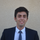cancel
Showing results for
Did you mean:Super User

## DAX Calculated % of a whole for Date

I have a calculated measure on my table which works perfectly to calculate each value as a % of the whole   Unit of Sale % = DIVIDE([Cummulative Units of Sale],CALCULATE([Cummulative Units of Sale],ALLSELECTED()))

However i want it to only calculate as a % of the month value i.e FY Month  so i changed it to this

Unit of Sale by Month % = DIVIDE([Cummulative Units of Sale],CALCULATE([Cummulative Units of Sale],FILTER('Date','Date'[TheDate]))) - this doesn't give the desires result it over inflates figures, so i obviously haven't a aclue what i am doing.

i actually want to slice it by the FY Year Month / Category but it doesn't like the string value in the filter where ive now put the date)

 FY M1 FY M2 Category 1 20% 30% Category 2 80% 70% 100% 100%

what is the correct DAX code for this?

If I took the time to answer your question and I came up with a solution, please mark my post as a solution and /or give kudos freely for the effort 🙂 Thank you!

Proud to be a Super User!

2 REPLIES 2MVP

I think you should use the Category rather than the Date Table as a filter.

The 20% in your screenshot means : [Cummulative Units of Sale] represents 20% of (Category 1 + Category 2) in FY M1

(If I understood well?).

So you have to ignore the filter context caused by the Category. To do so, use the ALL function.

Try this:

[Cummulative Units of Sale All Cat] = Calculate( [Cummulative Units of Sale] , All(Category) )

And Then:

[Unit of Sale by Month %] = Divide ( [Cummulative Units of Sale] , [Cummulative Units of Sale All Cat] )

And let us know if it fits your requirements !

Equally, I suggest you break your measures into several parts ( As I did for [Unit of Sale by Month %] by creating, first and separately, [Cummulative Units of Sale All Cat] measure).

Using interim measures makes it easier to read, understand and update the measure(s).

By the way, prefer the term measures to "calculated measures" which could confuse your colleagues with "calculated columns". Measures used to be called "calculated fields" in Excel 2013 but {fortunately} Measures term came back in Excel 2016/Power BI Desktop.Super User

i just changed what i have to category and it worked perfectly, i didnt use your statement so didn't test it but this is working great now!

My measures are already broken up for easy for understanding, thanks for the help, since im slicing on date i dont need to create a separate measure for it!

If I took the time to answer your question and I came up with a solution, please mark my post as a solution and /or give kudos freely for the effort 🙂 Thank you!

Proud to be a Super User!